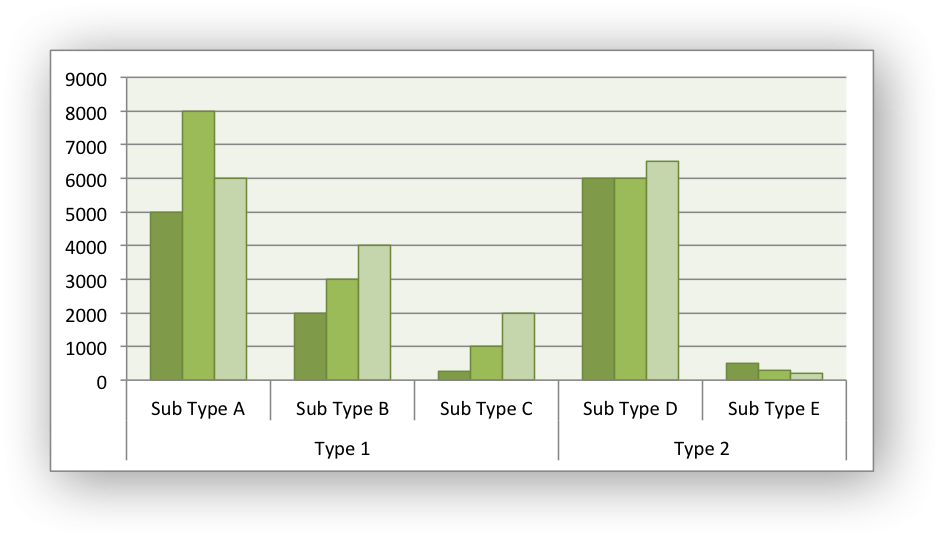libxlsxwriter
chart_clustered.c

Example of creating a clustered Excel chart where there are two levels of category on the X axis.

The categories in clustered charts are 2D ranges, instead of the more normal 1D ranges. The series are shown as formula strings for clarity but you can also use the a list syntax./*
* An example of a clustered category chart using the libxlsxwriter library.
*
* Copyright 2014-2021, John McNamara, jmcnamara@cpan.org
*
*/
#include "xlsxwriter.h"
/*
* Write some data to the worksheet.
*/
void write_worksheet_data(lxw_worksheet *worksheet, lxw_format *bold) {
worksheet_write_string(worksheet, 0, 0, "Types", bold);
worksheet_write_string(worksheet, 1, 0, "Type 1", NULL);
worksheet_write_string(worksheet, 4, 0, "Type 2", NULL);
worksheet_write_string(worksheet, 0, 1, "Sub Type", bold);
worksheet_write_string(worksheet, 1, 1, "Sub Type A", NULL);
worksheet_write_string(worksheet, 2, 1, "Sub Type B", NULL);
worksheet_write_string(worksheet, 3, 1, "Sub Type C", NULL);
worksheet_write_string(worksheet, 4, 1, "Sub Type D", NULL);
worksheet_write_string(worksheet, 5, 1, "Sub Type E", NULL);
worksheet_write_string(worksheet, 0, 2, "Value 1", bold);
worksheet_write_number(worksheet, 1, 2, 5000, NULL);
worksheet_write_number(worksheet, 2, 2, 2000, NULL);
worksheet_write_number(worksheet, 3, 2, 250, NULL);
worksheet_write_number(worksheet, 4, 2, 6000, NULL);
worksheet_write_number(worksheet, 5, 2, 500, NULL);
worksheet_write_string(worksheet, 0, 3, "Value 2", bold);
worksheet_write_number(worksheet, 1, 3, 8000, NULL);
worksheet_write_number(worksheet, 2, 3, 3000, NULL);
worksheet_write_number(worksheet, 3, 3, 1000, NULL);
worksheet_write_number(worksheet, 4, 3, 6000, NULL);
worksheet_write_number(worksheet, 5, 3, 300, NULL);
worksheet_write_string(worksheet, 0, 4, "Value 3", bold);
worksheet_write_number(worksheet, 1, 4, 6000, NULL);
worksheet_write_number(worksheet, 2, 4, 4000, NULL);
worksheet_write_number(worksheet, 3, 4, 2000, NULL);
worksheet_write_number(worksheet, 4, 4, 6500, NULL);
worksheet_write_number(worksheet, 5, 4, 200, NULL);
}
/*
* Create a worksheet with examples charts.
*/
int main() {
lxw_workbook *workbook = workbook_new("chart_clustered2.xlsx");
/* Add a bold format to use to highlight the header cells. */
/* Write some data for the chart. */
write_worksheet_data(worksheet, bold);
/*
* Configure the series. Note, that the categories are 2D ranges (from
* column A to column B). This creates the clusters. The series are shown
* as formula strings for clarity but you can also use variables with the
* chart_series_set_categories() and chart_series_set_values()
* functions. See the docs.
*/
"=Sheet1!\$A\$2:\$B\$6",
"=Sheet1!\$C\$2:\$C\$6");
"=Sheet1!\$A\$2:\$B\$6",
"=Sheet1!\$D\$2:\$D\$6");
"=Sheet1!\$A\$2:\$B\$6",
"=Sheet1!\$E\$2:\$E\$6");
/* Set an Excel chart style. */
chart_set_style(chart, 37);
/* Turn off the legend. */
/* Insert the chart into the worksheet. */
worksheet_insert_chart(worksheet, CELL("G3"), chart);
return workbook_close(workbook);
}
chart_set_style
void chart_set_style(lxw_chart *chart, uint8_t style_id)
Set the chart style type.
workbook_close
lxw_error workbook_close(lxw_workbook *workbook)
Close the Workbook object and write the XLSX file.
lxw_chart_series * chart_add_series(lxw_chart *chart, const char *categories, const char *values)
Add a data series to a chart.
workbook_new
lxw_workbook * workbook_new(const char *filename)
Create a new workbook object.
format_set_bold
void format_set_bold(lxw_format *format)
Turn on bold for the format font.
lxw_worksheet
Struct to represent an Excel worksheet.
Definition: worksheet.h:2107
lxw_format
Struct to represent the formatting properties of an Excel format.
Definition: format.h:358
lxw_chart
Struct to represent an Excel chart.
Definition: chart.h:1090
worksheet_insert_chart
lxw_error worksheet_insert_chart(lxw_worksheet *worksheet, lxw_row_t row, lxw_col_t col, lxw_chart *chart)
Insert a chart object into a worksheet.
lxw_workbook
Struct to represent an Excel workbook.
Definition: workbook.h:292
chart_legend_set_position
void chart_legend_set_position(lxw_chart *chart, uint8_t position)
Set the position of the chart legend.
worksheet_write_string
lxw_error worksheet_write_string(lxw_worksheet *worksheet, lxw_row_t row, lxw_col_t col, const char *string, lxw_format *format)
Write a string to a worksheet cell.
worksheet_write_number
lxw_error worksheet_write_number(lxw_worksheet *worksheet, lxw_row_t row, lxw_col_t col, double number, lxw_format *format)
Write a number to a worksheet cell.
CELL
#define CELL(cell)
Convert an Excel A1 cell string into a (row, col) pair.
Definition: utility.h:45
lxw_worksheet * workbook_add_worksheet(lxw_workbook *workbook, const char *sheetname)
Add a new worksheet to a workbook.